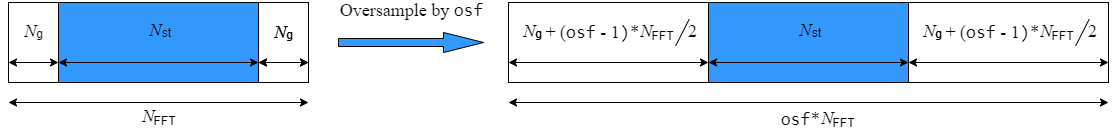# wlanNonHTOFDMInfo

OFDM information for non-HT transmission

## Syntax

``info = wlanNonHTOFDMInfo(field)``
``info = wlanNonHTOFDMInfo(field,bandwidth)``
``info = wlanNonHTOFDMInfo(field,cfg)``
``info = wlanNonHTOFDMInfo(___,OversamplingFactor=osf)``

## Description

example

````info = wlanNonHTOFDMInfo(field)` returns orthogonal frequency-division multiplexing (OFDM) information for the specified field of a non-high-throughput (non-HT) transmission.```

example

````info = wlanNonHTOFDMInfo(field,bandwidth)` specifies the channel bandwidth of the non-HT transmission.```

example

````info = wlanNonHTOFDMInfo(field,cfg)` specifies non-HT transmission parameters.```
````info = wlanNonHTOFDMInfo(___,OversamplingFactor=osf)` returns OFDM information for the specified oversampling factor. For more information about oversampling, see FFT-Based Oversampling.```

## Examples

collapse all

Get and display the OFDM information for the non-HT-Data field.

```info = wlanNonHTOFDMInfo('NonHT-Data'); disp(info);```
``` FFTLength: 64 SampleRate: 20000000 CPLength: 16 NumSubchannels: 1 NumTones: 52 ActiveFrequencyIndices: [52x1 double] ActiveFFTIndices: [52x1 double] DataIndices: [48x1 double] PilotIndices: [4x1 double] ```

OFDM demodulate the L-LTF in a non-HT transmission, then extract the data and pilot subcarriers.

Generate a WLAN waveform for a non-HT transmission.

```cfg = wlanNonHTConfig; bits = [1; 0; 0; 1]; waveform = wlanWaveformGenerator(bits,cfg);```

Obtain the field indices and extract the L-LTF.

```ind = wlanFieldIndices(cfg); rx = waveform(ind.LLTF(1):ind.LLTF(2),:);```

Perform OFDM demodulation on the L-LTF.

`sym = wlanLLTFDemodulate(rx,cfg);`

Get the OFDM information, then extract the data and pilot subcarriers.

```bandwidth = cfg.ChannelBandwidth; info = wlanNonHTOFDMInfo('L-LTF',bandwidth); data = sym(info.DataIndices,:,:); pilots = sym(info.PilotIndices,:,:);```

Configure and generate a non-HT time-domain waveform.

```cfg = wlanNonHTConfig('MCS',4); bits = randi([0 1],8*cfg.PSDULength,1,'int8'); waveform = wlanWaveformGenerator(bits,cfg);```

Transmit the waveform through an additive white Gaussian noise (AWGN) channel with a signal-to-noise ratio (SNR) of 30.

```snr = 30; rxWaveform = awgn(waveform,snr);```

Extract the non-HT Data field from the received waveform.

```field = 'NonHT-Data'; ind = wlanFieldIndices(cfg,field); rx = rxWaveform(ind(1):ind(2),:);```

Recover the frequency-domain signal by OFDM demodulating the time-domain data signal.

`sym = wlanNonHTOFDMDemodulate(rx,field,cfg);`

Extract the data subcarriers from the demodulated signal.

```info = wlanNonHTOFDMInfo(field,cfg); sym = sym(info.DataIndices,:,:);```

Recover the PSDU and confirm that it matches the transmitted PSDU.

```noiseVarEst = 10^(-snr/10); psdu = wlanNonHTDataBitRecover(sym,noiseVarEst,cfg); isequal(bits,psdu)```
```ans = logical 1 ```

## Input Arguments

collapse all

Field for which the function returns OFDM information, specified as one of these values.

• `'L-LTF'` – Return OFDM information for the legacy long training field (L-LTF).

• `'L-SIG'` – Return OFDM information for the legacy signal (L-SIG) field.

• `'NonHT-Data'` – Return OFDM information for the non-HT Data field.

Data Types: `char` | `string`

Channel bandwidth of PPDU transmission, specified as one of these values.

• `'CBW5'` — Channel bandwidth of 5 MHz

• `'CBW10'` — Channel bandwidth of 10 MHz

• `'CBW20'` — Channel bandwidth of 20 MHz

• `'CBW40'` — Channel bandwidth of 40 MHz for non-HT duplicate

• `'CBW80'` — Channel bandwidth of 80 MHz for non-HT duplicate

• `'CBW160'` — Channel bandwidth of 160 MHz for non-HT duplicate

Data Types: `char` | `string`

Non-HT transmission parameters, specified as a `wlanNonHTConfig` object. Because this function supports only OFDM modulation, you must set the `Modulation` property of this input to `'OFDM'`.

Oversampling factor, specified as a scalar greater than or equal to 1. The oversampled cyclic prefix length must be an integer number of samples.

Data Types: `single` | `double` | `int8` | `int16` | `int32` | `int64` | `uint8` | `uint16` | `uint32` | `uint64`

## Output Arguments

collapse all

OFDM information, returned as a structure containing these fields.

NameValuesDescriptionData Types
`FFTLength`Positive integerLength of the fast Fourier transform (FFT)`double`
`SampleRate`Positive scalarSample rate of the waveform`double`
`CPLength`Positive integer

Cyclic prefix length, in samples

`double`
`NumTones`Nonnegative integer

Number of active subcarriers

`double`
`NumSubchannels`Positive integerNumber of 20-MHz subchannels`double`
`ActiveFrequencyIndices`Column vector of integers in the interval [-`FFTLength`/2, (`FFTLength`/2 – 1)]Indices of active subcarriers. Each element of this field is the index of an active subcarrier, such that the direct current (DC) or null subcarrier is at the center of the frequency band.`double`
`ActiveFFTIndices`Column vector of integers in the interval [1, `FFTLength`]Indices of active subcarriers within the FFT`double`
`DataIndices`Column vector of integers in the interval [1, `NumTones`]Indices of data within the active subcarriers`double`
`PilotIndices`Column vector of integers in the interval [1, `NumTones`]Indices of pilots within the active subcarriers`double`

Data Types: `struct`

## Algorithms

collapse all

### FFT-Based Oversampling

An oversampled signal is a signal sampled at a frequency that is higher than the Nyquist rate. WLAN signals maximize occupied bandwidth by using small guardbands, which can pose problems for anti-imaging and anti-aliasing filters. Oversampling increases guardband width relative to the total signal bandwidth, thereby increasing the number of samples in the signal.

This function performs oversampling by using a larger IFFT and zero pad when generating an OFDM waveform. This diagram shows the oversampling process for an OFDM waveform with NFFT subcarriers comprising Ng guardband subcarriers on either side of Nst occupied bandwidth subcarriers.## Version History

Introduced in R2019a